Next: Worked example 5.4: Roller Up: Conservation of energy Previous: Worked example 5.2: Dragging

## Worked example 5.3: Stretching a spring

Question: The force required to slowly stretch a spring varies from 0N to 105N as the spring is extended by 13cm from its unstressed length. What is the force constant of the spring? What work is done in stretching the spring? Assume that the spring obeys Hooke's law.

Answer: The forcethat the spring exerts on whatever is stretching it is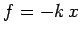, whereis the force constant, andis the extension of the spring. The minus sign indicates that the force acts in the opposite direction to the extension. Since the spring is stretched slowly, the force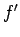which must be exerted on it is (almost) equal and opposite to. Hence,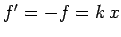. We are told that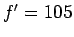N when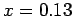m. It follows that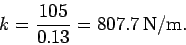The work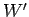done by the external force in extending the spring from 0 tois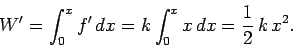Hence,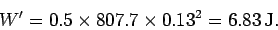Next: Worked example 5.4: Roller Up: Conservation of energy Previous: Worked example 5.2: Dragging
Richard Fitzpatrick 2006-02-02